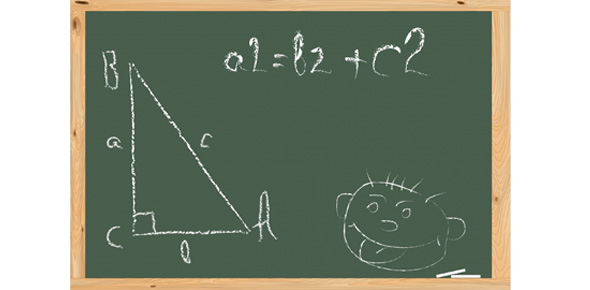# Section 8.3 - The Pythagorean Theorem

5 Questions | Total Attempts: 245SettingsTo all the mathematics lovers out there who indulge in different formula and books for both high and low levels of study take this quiz. It is specifically designed to help you find out how much you know about the Pythagorean Theorem.

Related Topics
• 1.
What is the measure of the missing length?
• A.

55 m

• B.

57 m

• C.

63 m

• D.

71 m

• 2.
Ms Lange drove about 150 km east from La sarre, to Senneterre, Quebec.She drove about another 75 km north to Lebel-sur-Quevillon.  What is the approximate air distance from La Sarre to Lebel-sur-Quevillon, Quebec?
• A.

160 km

• B.

168 km

• C.

175 km

• D.

225 km

• 3.
What is the area of Square C?
• A.

90 cm^2

• B.

809 cm^2

• C.

2009 cm^2

• D.

3969 cm^2

• 4.
What is the measure of the missing length?
• A.

13 m

• B.

14 m

• C.

15 m

• D.

16 m

• 5.
What is the measure of the diagonal of the square?
• A.

18.9 mm

• B.

60.0 mm

• C.

75.0 mm

• D.

84.9 mm